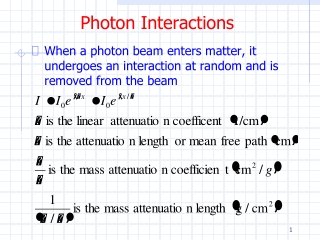DownloadDownload PresentationPhoton Interactions

# Photon Interactions

Download Presentation## Photon Interactions

- - - - - - - - - - - - - - - - - - - - - - - - - - - E N D - - - - - - - - - - - - - - - - - - - - - - - - - - -
##### Presentation Transcript

1. Photon Interactions • When a photon beam enters matter, it undergoes an interaction at random and is removed from the beam

2. Photon Interactions

3. Photon Interactions • Notes • l is the average distance a photon travels before interacting • l is also the distance where the intensity drops by a factor of 1/e = 37% • For medical applications, HVL is frequently used • Half Value Layer • Thickness needed to reduce the intensity by ½ • Gives an indirect measure of the photon energies of a beam (under the conditions of a narrow-beam geometry) • In shielding calculations, you will see TVL used a lot

4. Beam Hardening

5. Photon Interactions

6. Photon Interactions • What is a cross section? • What is the relation of m to the cross section s for the physical process?

7. Cross Section • Consider scattering from a hard sphere • What would you expect the cross section to be? α θ α R b α

8. Cross Section • The units of cross section are barns • 1 barn (b) = 10-28m2 = 10-24cm2 • The units are area. One can think of the cross section as the effective target area for collisions. We sometimes take σ=πr2

9. Cross Section • One can find the scattering rate by

10. Cross Section • For students working at collider accelerators

11. Photon Interactions • In increasing order of energy the relevant photon interaction processes are • Photoelectric effect • Rayleigh scattering • Compton scattering • Photonuclear absorption • Pair production

12. Photon Interactions • Relative importance of the photoelectric effect, Compton scattering, and pair production versus energy and atomic number Z

13. Photoelectric Effect • An approximate expression for the photoelectric effect cross section is • What’s important is that the photoelectric effect is important • For high Z materials • At low energies (say < 0.1 MeV)

14. Photoelectric Effect • More detailed calculations show

15. Photon Interactions • Typical photon cross sections

16. Photoelectric Effect • The energy of the (photo)electron is • Binding energies for some of the heavier elements are shown on the next page • Recall from the Bohr model, the binding energies go as

17. Photoelectric Effect

18. Photoelectric Effect • The energy spectrum looks like • This is because at these photon/electron energies the electron is almost always absorbed in a short distance • As are any x-rays emitted from the ionized atom

19. Photoelectric Effect and X-rays • PE proportionality to Z5 makes diagnostic x-ray imaging possible • Photon attenuation in • Air – negligible • Bone – significant (Ca) • Soft tissue (muscle e.g.) – similar to water • Fat – less than water • Lungs – weak (density) • Organs (soft tissue) can be differentiated by the use of barium (abdomen) and iodine (urography, angiography)

20. Photoelectric Effect and X-rays • Typical diagnostic x-ray spectrum • 1 anode, 2 window, 3 additional filters

21. Photon Interactions • Sometimes easy to loose sight of real thickness of material involved

22. Photon Interactions • X-ray contrast depends on differing attenuation lengths

23. Photoelectric Effect • Related to kerma (Kinetic Energy Released in Mass Absorption) and absorbed dose is the fraction of energy transferred to the photoelectron • As we learned in a previous lecture, removal of an inner atomic electron is followed by x-ray fluorescence and/or the ejection of Auger electrons • The latter will contribute to kerma and absorbed dose

24. Photoelectric Effect • Thus a better approximation of the energy transferred to the photoelectron is • We can then define e.g.

25. Photoelectric Effect • Fluorescence yield Y for K shell

26. Cross Section • dΩ=dA/r2=sinθdθdφ

27. Cross Section

28. Cross Section • If a particle arrives with an impact parameter between b and b+db, it will emerge with a scattering angle between θ and θ+dθ • If a particle arrives within an area of dσ, it will emerge into a solid angle dΩ

29. Cross Section • From the figure on slide 7 we see • This is the relation between b and θ for hard sphere scattering

30. Cross Section • We have • And the proportionality constant dσ/dΩ is called the differential cross section

31. Cross Section • Then we have • And for the hard sphere example

32. Cross Section • Finally • This is just as we expect • The cross section formalism developed here is the same for any type of scattering (Coulomb, nuclear, …) • Except in QM, the scattering is not deterministic

33. Cross Section • We have • And the proportionality constant dσ/dΩ is called the differential cross section • The total cross section σ is just